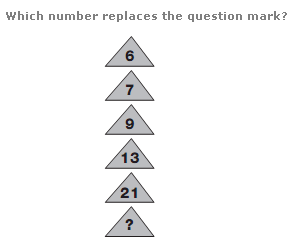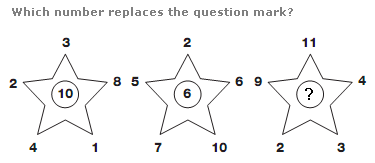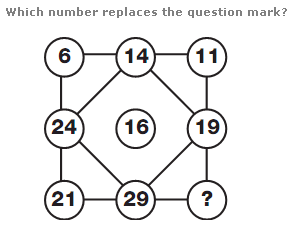# Puzzles - Number puzzles

### Exercise :: Number puzzlesAnswer : 37 Explanation : As you move down the diagram, double each number and subtract 5 to give the next number.Answer : 17 Explanation : In each star, the number in the centre equals the difference between the sum of the even numbers and the sum of the odd numbers around the points of the star.Answer : 26 Explanation : Starting on the top left and moving to the right, then down one row and to the left, then down to the bottom row and to the right, numbers alternately add 8 then subtract 3.